# AI 安全之对抗样本入门 (2)：深度学习基础知识 1.1.1&1.1.2(数据预处理)

(定义网络结构)

$y=w*x+b$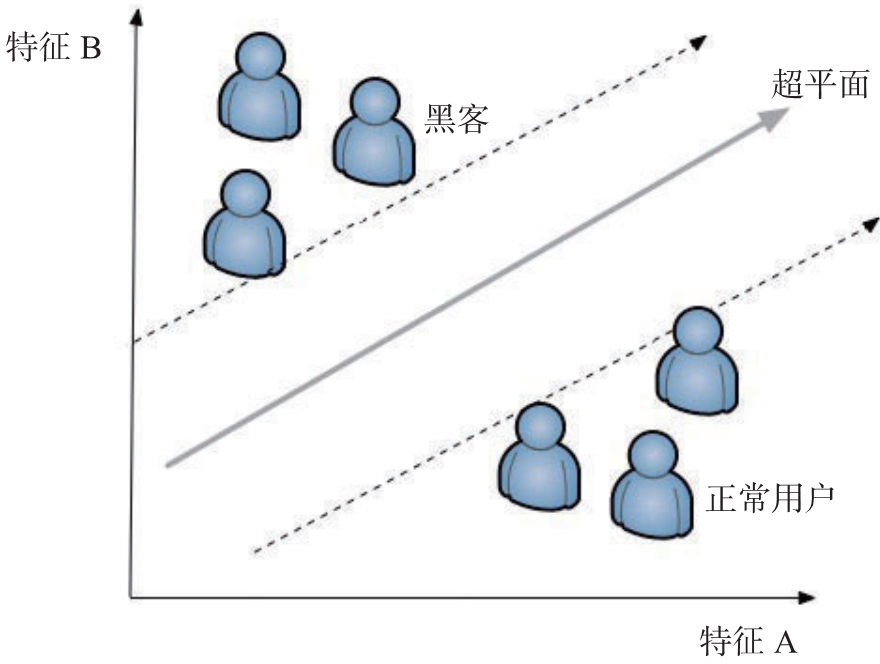1. Dense 层

Dense 层是最常见的网络层，用于构建一个全连接。一个典型的全连接结构由输入、求和、激活、权重矩阵、偏置和输出组成，如图 1-2 所示，训练的过程就是不断获得最优的权重矩阵和偏置（bias）的过程。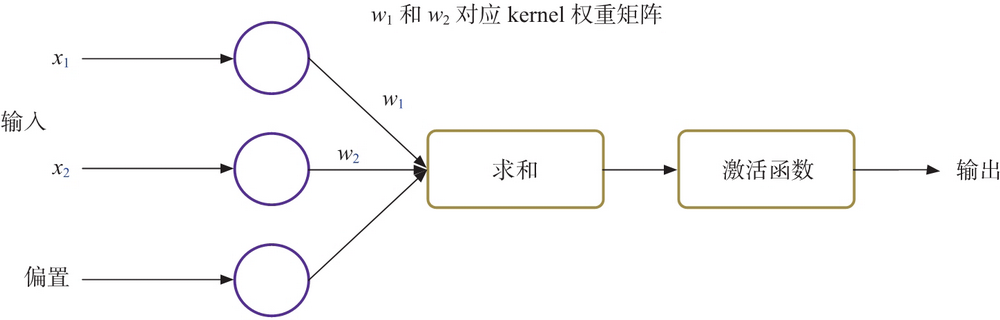keras.layers.core.Dense(units, activation=None, use_bias=True,kernel_initializer='glorot_uniform', bias_initializer='zeros',kernel_regularizer=None, bias_regularizer=None, activity_regularizer=None,kernel_constraint=None, bias_constraint=None)

• units 表示隐藏层节点数。
• activation（激活函数）详细介绍请参见 Activation 层的相关内容。
• use_bias 表示是否使用偏置。
1. Activation 层

Actiration 层对一个层的输出施加激活函数，常见的激活函数包括以下几种。

（1）relu

relu 函数当输入小于 0 时为 0，当输入大于 0 时等于输入。使用代码绘制 relu 的图像，获得图像（见图 1-3）。

def relu(x):    if x > 0:        return x    else:        return 0def func4():    x = np.arange(-5.0, 5.0, 0.02)    y=[]    for i in x:        yi=relu(i)        y.append(yi)    plt.xlabel('x')    plt.ylabel('y relu(x)')    plt.title('relu')    plt.plot(x, y)    plt.show()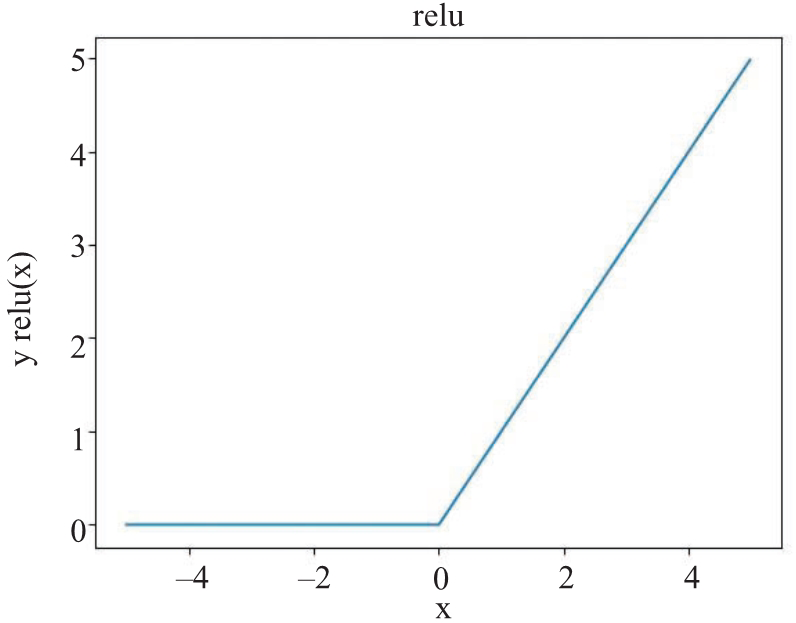（2）leakyrelu

leakyrelu 函数是从 relu 函数发展而来的，当输入小于 0 时为输入乘以一个很小的系数，比如 0.1，当输入大于 0 时等于输入。使用代码绘制 leakyrelu 的图像，获得图像（见图 1-4）。

def leakyrelu(x):    if x > 0:        return x    else:        return x*0.1def func5():    x = np.arange(-5.0, 5.0, 0.02)    y=[]    for i in x:        yi=leakyrelu(i)        y.append(yi)    plt.xlabel('x')    plt.ylabel('y leakyrelu(x)')    plt.title('leakyrelu')    plt.plot(x, y)    plt.show()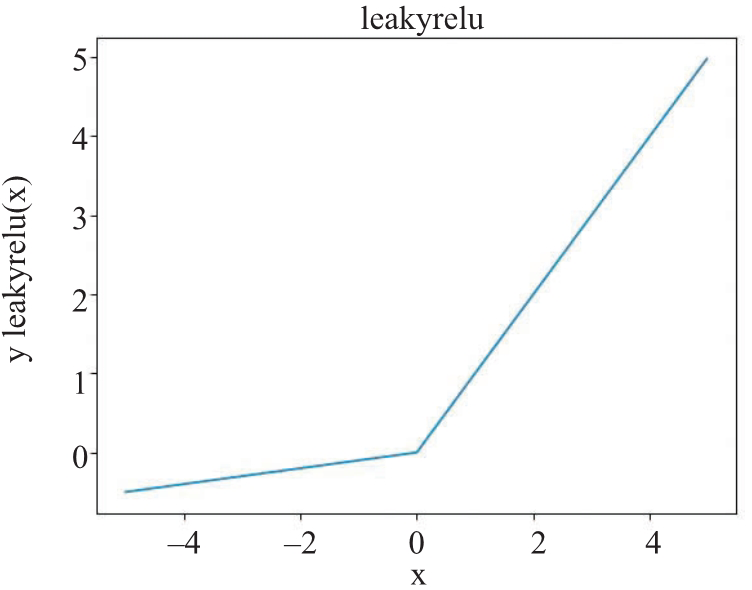（3）tanh

tanh 也称为双切正切函数，取值范围为 [–1, 1]。tanh 在特征相差明显时的效果会很好，在循环过程中会不断扩大特征效果。tanh 的定义如下：

$f(x)=\frac{e^x-e^{-x}}{e^x+e^{-x}}$

x = np.arange(-5.0, 5.0, 0.02)y=(np.exp(x)-np.exp(-x))/(np.exp(x)+np.exp(-x))plt.xlabel('x')plt.ylabel('y tanh(x)')plt.title('tanh')plt.plot(x, y)plt.show()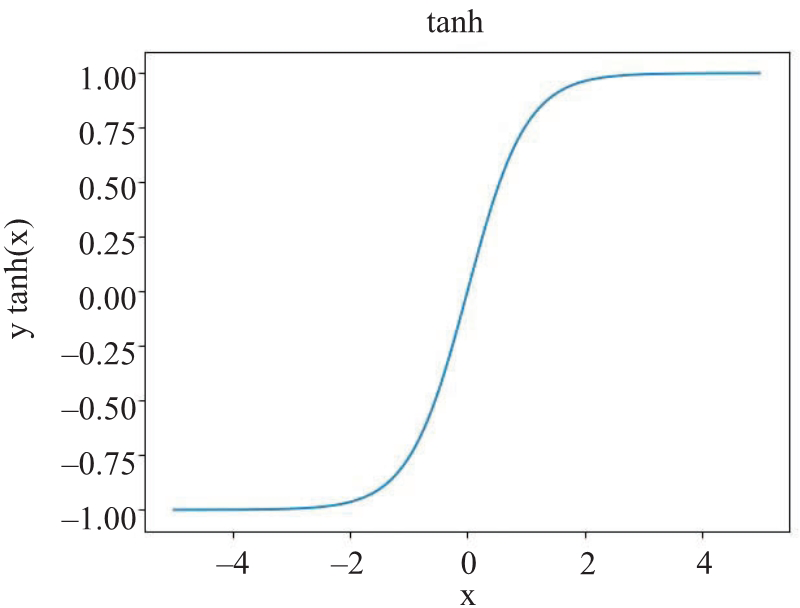（4）sigmoid

sigmoid 可以将一个实数映射到 (0, 1) 的区间，可以用来做二分类。sigmoid 的定义如下：

$f(x)=\frac{1}{1+e^{-x}}$

x = np.arange(-5.0, 5.0, 0.02)y=1/(1+np.exp(-x))plt.xlabel('x')plt.ylabel('y sigmoid(x)')plt.title('sigmoid')plt.plot(x, y)plt.show()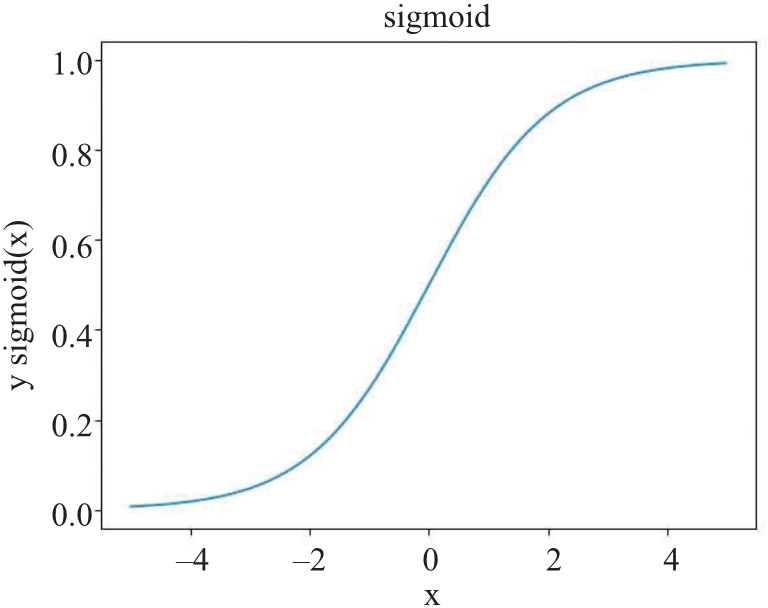Activation 层可以单独使用，也可以作为其他层的参数，比如创建一个输入大小为 784，节点数为 32，激活函数为 relu 的全连接层的代码为：

model.add(Dense(32, input_shape=(784,)))model.add(Activation('relu'))

model.add(Dense(32, activation='relu'，input_shape=(784,)))
1. Dropout 层

keras.layers.core.Dropout(rate, noise_shape=None, seed=None)

1. Embedding 层

Embedding 层负责将输入的向量按照一定的规则改变维度，有点类似于 Word2Vec 的处理方式，把词可以映射到一个指定维度的向量中，其函数定义如下：

keras.layers.Embedding(input_dim, output_dim,embeddings_initializer='uniform', embeddings_regularizer=None,activity_regularizer=None, embeddings_constraint=None, mask_zero=False,input_length=None)

• input_dim：输入的向量的维度。
• output_dim：输出的向量的维度。
• embeddings_initializer：初始化的方式，通常使用 glorot_normal 或者 uniform。
1. Flatten 层

Flatten 层用来将输入压平，即把多维的输入一维化。

1. Permute 层

Permute 层将输入的维度按照给定模式进行重排。一个典型场景就是在 Keras 处理图像数据时，需要根据底层是 TensorFlow 还是 Theano 调整像素的顺序。在 TensorFlow 中图像保存的顺序是 (width, height, channels) 而在 Theano 中则为 (channels, width, height)，比如 MNIST 图像，在 TensorFlow 中的大小就是 (28,28,1)，而在 Theano 中是 (1, 28, 28)。示例代码如下：

if K.image_dim_ordering() == 'tf':    # (width, height, channels)    model.add(Permute((2, 3, 1), input_shape=input_shape))elif K.image_dim_ordering() == 'th':    # (channels, width, height)    model.add(Permute((1, 2, 3), input_shape=input_shape))else:    raise RuntimeError('Unknown image_dim_ordering.')
1. Reshape 层

Reshape 层用于将输入 shape 转换为特定的 shape。函数定义如下代码所示：

keras.layers.core.Reshape(target_shape)